Math resources Geometry Volume

Volume of a pyramid

# Volume of a pyramid

Here you will learn about the volume of a pyramid, including how to find the volume and how to apply it to real world scenarios.

Students first learn about the volume of a pyramid with their work in geometry in the 7 th grade and expand that knowledge as they progress through high school.

## What is the volume of a pyramid?

The volume of a pyramid is how much space there is inside the pyramid. Volume is measured in cubic units.

For example, the number of small red balls inside the pyramid represents the approximate volume of the pyramid because it represents the space inside the pyramid.

Did you know that the volume of a pyramid is the same as \cfrac{1}{3} \, the volume of a prism with the same height and base?

Let’s look at an example by comparing the volumes of a pyramid and a prism with the same heights (perpendicular height) and the same square bases.

Recall how to find the volume of a prism,

V=\text { area of the base } \cdot \text { height }

The base of the prism is a square with side length of 4 units.

The area of the base is 4 \times 4=16

The height of the prism is 9 units, so the volume is:

\begin{aligned}& V=(16) \times 9 \\\\ & V=144\end{aligned}

The volume of the prism is 144 \text {~units}^3.

The volume of a pyramid is:

V=\cfrac{1}{3} \, \times \text { area of the base } \times \text { height }

The base of the pyramid is a square, the same as the prism, with side lengths of 4 units.

The area of the base is 4 \times 4=16

The height of the pyramid is 9 units, so the volume is:

\begin{aligned}& V=\cfrac{1}{3} \times 16 \times 9 \\\\ & V=48\end{aligned}

The volume of the pyramid is 48 \text {~units}^3.

\cfrac{1}{3} \times 144=48 → one-third the volume of the prism is the volume of the pyramid

To find the volume of a pyramid, you can always use:

V=\cfrac{1}{3} \times \text { area of the base } \times \text { height }

You can also use the formula:

V=\cfrac{1}{3} \, Bh

B = \text { area of the base}

h = \text { perpendicular height of the 3D object }

### What is the volume of a pyramid?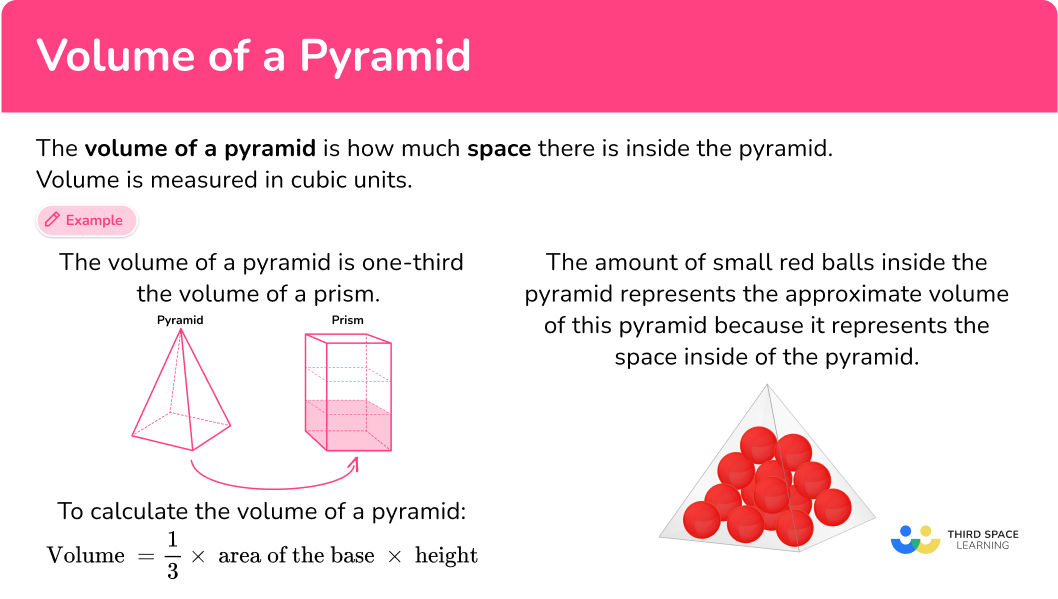## Common Core State Standards

How does this apply to 7 th grade math?

• Grade 7 – Geometry (7.G.B.6)
Solve real-world and mathematical problems involving area, volume and surface area of two- and three-dimensional objects composed of triangles, quadrilaterals, polygons, cubes, and right prisms.

## How to calculate the volume of a pyramid

In order to calculate the volume of a pyramid:

1. Calculate the area of the base.
2. Substitute values into the formula and solve.
3. Write the answer, including the units.

## Volume of a pyramid examples

### Example 1: Pyramid real world

The Great Pyramid in Egypt is 137 \, meters in height and has a square base that has an area of 52,900 \text { meters }{ }^2. What is the volume of the Great Pyramid rounded to the nearest whole number?

1. Calculate the area of the base.

The base of the Great Pyramid is a square. The area of the base is given to be 52,900 \text { meters}^2.

2Substitute values into the formula and solve.

The height of the Great Pyramid is 137 \, meters.

To find volume of a pyramid, you can use:

\begin{aligned}& V=\cfrac{1}{3} \times \text { area of base } \times \text { height } \\\\ & V=\cfrac{1}{3} \times52,900 \times 137 \\\\ & V=2,415,766.67 \end{aligned}

Rounded to the nearest whole number is 2,415,767.

3Write the answer, including the units.

The volume of the Great Pyramid is about 2,415,767 \text { meters }^3.

### Example 2: calculate volume of a square pyramid

Find the volume of the pyramid.

The base of the pyramid is a square with side length 6 \, cm.

Area of a square is b \times h

\begin{aligned}& \text { Area of base }=6 \times 6 \\\\ & \text { Area of base }=36\end{aligned}

To find the volume of a pyramid you can use:

\begin{aligned}& V=\cfrac{1}{3} \times \text { area of the base } \times \text { height } \\\\ & \text { area of the base }=36 \\\\ & \text { height }=8 \\\\ & V=\cfrac{1}{3} \times 36 \times 8 \\\\ & V=96\end{aligned}

The volume of the pyramid is 96 \mathrm{~cm}^3.

### Example 3: calculate volume of a pyramid

Find the volume of the rectangular pyramid.

The base of the pyramid is a rectangle with side lengths 1 inch and 2 inches.

Area of a rectangle is b \times h

\begin{aligned}& \text { Area of base }=1 \times 2 \\\\ & \text { Area of base }=2\end{aligned}

To find the volume of a pyramid you can use:

\begin{aligned} & V=\cfrac{1}{3} \times \text { area of the base } \times \text { height } \\\\ & \text { area of the base }=2 \\\\ & \text { height }=2 \\\\ & V=\cfrac{1}{3} \times 2 \times 2 \\\\ & V=\cfrac{4}{3}=1 \cfrac{1}{3}\end{aligned}

The volume of the pyramid is 1 \cfrac{1}{3} \text { inches}^3.

### Example 4: finding height of a pyramid

The volume of a triangular pyramid that has a triangular base with an area of 33 \text { units}^2 and a perpendicular height of 15 \, units.

The base is a triangle with an area of 33 \text { units}^2.

To find the volume of a pyramid you can use:

\begin{aligned}& V=\cfrac{1}{3} \times \text { area of the base } \times \text { height } \\\\ & \text { area of the base }=33 \\\\ & \text { height }=15 \\\\ & V=\cfrac{1}{3} \times 33 \times 15 \\\\ & V=165\end{aligned}

The volume of the pyramid is 165 \text { units}^3.

### Example 5: hexagonal base pyramid

Calculate the volume of this pyramid.

The base of the pyramid is a hexagon with an area of 14.2 \mathrm{~cm}^2.

To find the volume of a pyramid you can use:

\begin{aligned}& V=\cfrac{1}{3} \times \text { area of the base } \times \text { height } \\\\ & \text { area of the base }=14.2 \\\\ & \text { height }=5.1 \\\\ & V=\cfrac{1}{3} \times 14.2 \times 5.1 \\\\ & V=24.14\end{aligned}

The volume of the hexagonal pyramid is 24.14 \mathrm{~cm}^3.

### Example 6: calculating the height

Calculate the height of a rectangular pyramid with volume 40 \mathrm{~cm}^3 and base area 12 \mathrm{~cm}^2.

The area of the base of the pyramid is 12 \mathrm{~cm}^2.

Using the formula for the volume of a pyramid:

\begin{aligned} & V =\cfrac{1}{3} \times \text { area of the base } \times \text { height } \\\\ & \text {area of the base } =12 \\\\ & volume = 40 \\\\ & height = \, ? \end{aligned}

\begin{aligned} 40 & =\cfrac{1}{3} \times 12 \times h \\\\ 40 & =4 \times h \\\\ 40 & =4 h \\\\ \cfrac{40}{4} & =\cfrac{4 h}{4} \\\\ 10 & =h\end{aligned}

The height of the pyramid is 10 \mathrm{~cm}.

### Teaching tips for volume of a pyramid

• Provide opportunities for students to work with manipulatives of 3D objects so that they can gain a sense of what volume means in relation to the object.

• Infusing activities where students can see patterns in numbers to formulate understanding is essential for deep understanding. For example, understanding that the volume of a pyramid is \cfrac{1}{3} the volume of a prism.

• Worksheets have a place in the classroom for practicing skills. However, include real world problems that incorporate the volume of pyramids so that students can see the relevance of the concept in the world around them.

### Easy mistakes to make

• The height is the perpendicular height
Sometimes when you are provided with several dimensions of 3D objects, be sure that you are reading the perpendicular height dimension correctly.
For example, in the pyramid pictured below, 6 \, cm is the slant height and 4 \, cm is the perpendicular height.
The perpendicular height is needed to find the volume of a pyramid whereas the slant height is needed for surface area of a pyramid.

• Writing the incorrect units or forgetting to include the units
Always include units when recording a measurement. Volume is measured in cubic units.
For example, \mathrm{mm}^3, \mathrm{~cm}^3, \mathrm{~m}^3, etc.

• Base is not always a square
Like prisms, pyramids can have any polygon as a base and more importantly, if the base is a quadrilateral, you cannot assume that it is a square. Always check for the base dimensions when working with area.

### Practice volume of a pyramid questions

1. Calculate the volume of the pyramid below.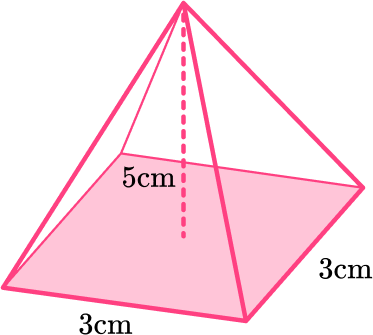45 \text{ cm}^315 \text{ cm}^311 \text{ cm}^330 \text{ cm}^3To find the volume of a pyramid:

V=\cfrac{1}{3} \times \text { area of the base } \times \text { height }

The base of the pyramid is a square with side length 3 \, cm.

\begin{aligned}& \text { Area of base }=3 \times 3=9 \\\\ & \text { height }=5 \mathrm{~cm} \\\\ & V=\cfrac{1}{3} \times \text { area of the base } \times \text { height } \\\\ & V=\cfrac{1}{3} \times 9 \times 5 \\\\ & V=15 \end{aligned}

The volume of the pyramid is   15 \mathrm{~cm}^3.

2. The heights of the prism and pyramid are equal, and the area of the bases is equal. If the volume of the prism is 96 \text{ units}^3, what is the volume of the pyramid?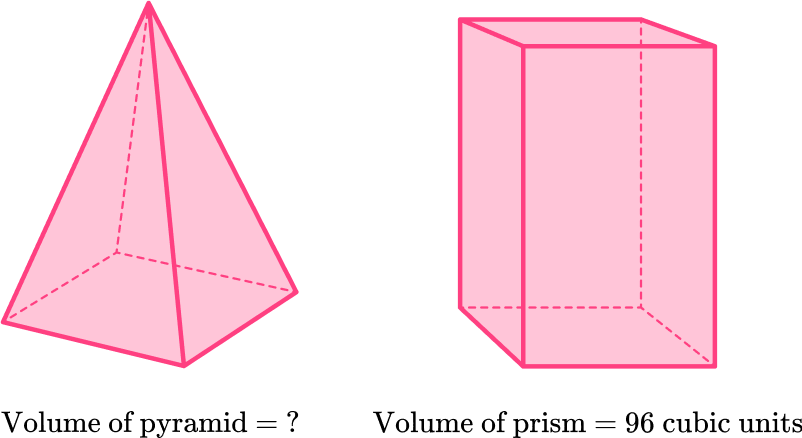48 \text { units}^3288 \text { units}^396 \text { units}^332 \text { units}^3The volume of a pyramid is \cfrac{1}{3} the volume of a prism with the same height and base area.

Since the volume of the prism is 96 you can either multiply 96 by \cfrac{1}{3} or divide 96 by 3 to get the volume of the pyramid.

96 \times \cfrac{1}{3}=32 \, or \, 96 \div 3=32

3. Calculate the volume of the pyramid below.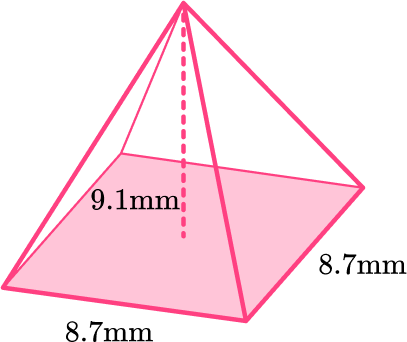229.5 \text{ mm}^3668.8 \text{ cm}^3229.6 \text{ mm}^3229.6 \text{ cm}^3To find the volume of a pyramid:

V=\cfrac{1}{3} \times \text { area of the base } \times \text { height }

The base of the pyramid is a square with side length 8.7 \, cm.

\begin{aligned}& \text { Area of base }=8.7 \times 8.7=75.69 \\\\ & \text { height }=9.1 \\\\ & V=\cfrac{1}{3} \times \text { area of the base } \times \text { height } \\\\ & V=\cfrac{1}{3} \times 75.69 \times 9.1 \\\\ & V=229.593\end{aligned}

The volume of the pyramid rounded to the nearest tenth is 229.6 \text{ mm}^3 .

4. Find the volume of a rectangular pyramid.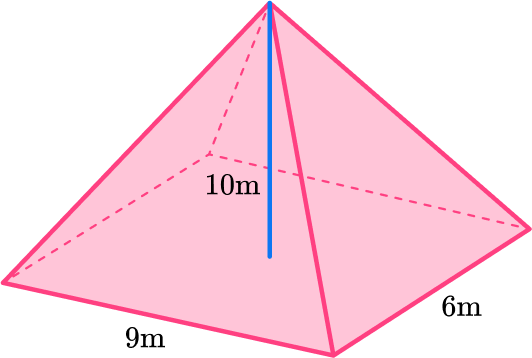180 \text{ m}^3120 \text{ m}^3270 \text{ m}^3540 \text{ m}^3To find the volume of a pyramid:

V=\cfrac{1}{3} \times \text { area of the base } \times \text { height }

The base of the pyramid is a rectangle with side lengths 9 \, m and 6 \, m.

\begin{aligned}& \text { Area of base }=9 \times 6=54 \\\\ & \text { height }=10 \\\\ & V=\cfrac{1}{3} \times \text { area of the base } \times \text { height } \\\\ & V=\cfrac{1}{3} \times 54 \times 10 \\\\ & V=180\end{aligned}

The volume of the rectangular pyramid is 180 \mathrm{~m}^3.

5. Find the volume of the triangular pyramid.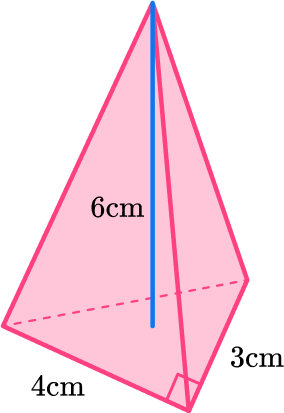24 \mathrm{~cm}^312 \mathrm{~cm}^318 \mathrm{~cm}^336 \mathrm{~cm}^3To find the volume of a pyramid:

V=\cfrac{1}{3} \times \text { area of the base } \times \text { height }

The base of the pyramid is a triangle.

The area of a triangle is:   \cfrac{1}{2} \times b \times h

\begin{aligned}& \text { Area of base }=\cfrac{1}{2} \times 3 \times 4 \\\\ & \text { Area of base }=6 \\\\ & \text { height }=6 \\\\ & V=\cfrac{1}{3} \times \text { area of the base } \times \text { height } \\\\ & V=\cfrac{1}{3} \times 6 \times 6 \\\\ & V=12\end{aligned}

The volume of the triangular pyramid is 12 \mathrm{~cm}^3.

6. Find the height of the pyramid if the volume is 144 \, m^3 and the area of the base is 18 \, m^3.

24 \mathrm{~cm}25 \mathrm{~cm}8 \mathrm{~cm}18 \mathrm{~cm}Use the formula:

V=\cfrac{1}{3} \times \text { area of the base } \times \text { height }

Substitute the known values into the equation.

V= 144

\text{Area of base } = 18

\begin{aligned}& 144=\cfrac{1}{3} \times 18 \times h \\\\ & 144=6 \times h \\\\ & 144=6 h \\\\ & \cfrac{144}{6}=\cfrac{6 h}{6} \\\\ & 24=h\end{aligned}

The height of the pyramid is 24 \mathrm{~cm}.

## Volume of pyramid FAQs

Do all pyramids have square bases?

No, there are many different types of pyramids that can have any polygonal base. For example, triangular pyramids have triangle bases, hexagonal pyramids have hexagon bases, square pyramids have square bases, rectangular pyramids have rectangular bases, Pentagonal pyramids have pentagonal bases, etc..

What is a polyhedron?

A polyhedron is a 3D object that is made up of polygonal sides.

How can you tell the difference between the slant height and the perpendicular height?

The slant height of a pyramid is the height of the triangular faces of the pyramid. The perpendicular height is the distance from the apex (top vertex) of the pyramid to the center of the base of the pyramid.

What is a regular pyramid?

A regular pyramid is a pyramid that has a regular polygon as its base.

How do you find the volume of a cone?

You find the volume of a cone similarly to how you find the volume of a pyramid. Take one-third of the product of the area of the circular base and the height of the cone.

## Still stuck?

At Third Space Learning, we specialize in helping teachers and school leaders to provide personalized math support for more of their students through high-quality, online one-on-one math tutoring delivered by subject experts.

Each week, our tutors support thousands of students who are at risk of not meeting their grade-level expectations, and help accelerate their progress and boost their confidence.